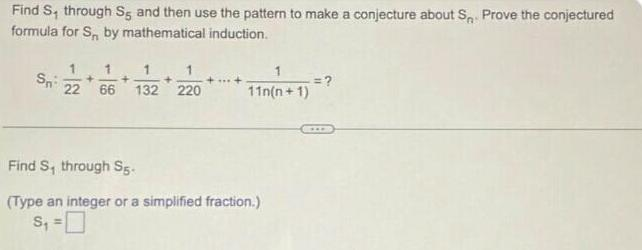Question:

# Find S, through S, and then use the pattern to make a

Last updated: 8/10/2022Find S, through S, and then use the pattern to make a conjecture about S, Prove the conjectured formula for S, by mathematical induction. Sn:1/22+1/66+1/132+1/220+...+1/11n(n+1) Find S, through S5- (Type an integer or a simplified fraction.) S₁ =